# Chapter 7 MULTIDIMENSIONAL ARRAYS Lecture notes for computer

• Slides: 14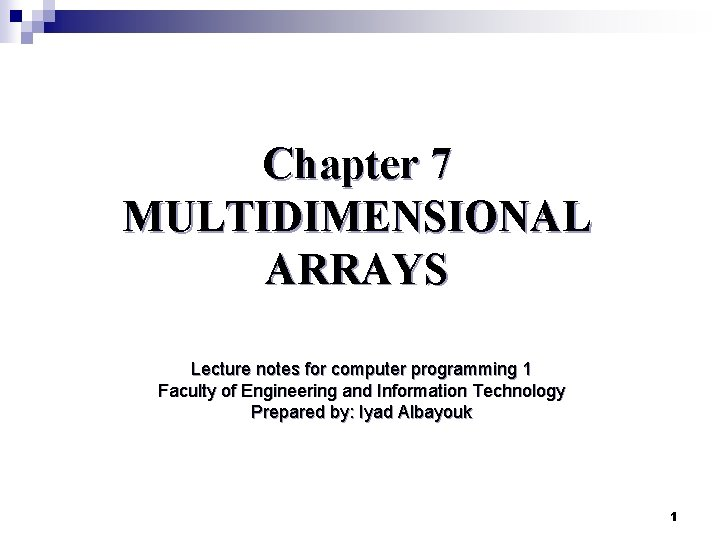Chapter 7 MULTIDIMENSIONAL ARRAYS Lecture notes for computer programming 1 Faculty of Engineering and Information Technology Prepared by: Iyad Albayouk 1Introduction n Data in a table or a matrix can be represented using a two-dimensional array. For example: 2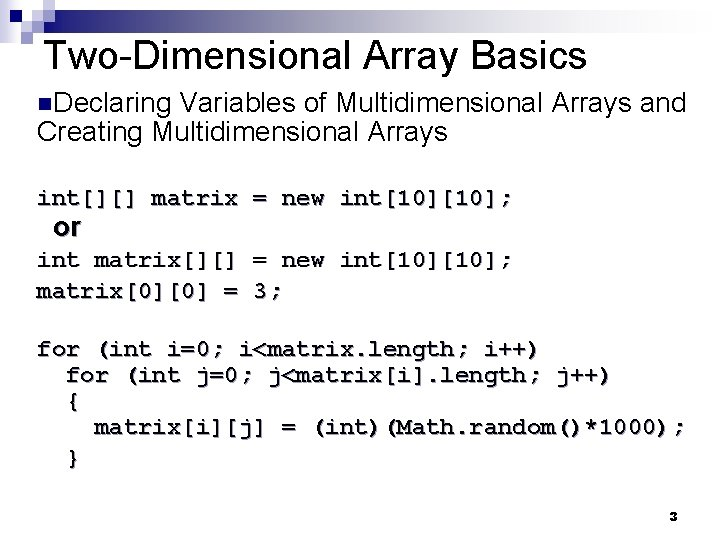Two-Dimensional Array Basics n. Declaring Variables of Multidimensional Arrays and Creating Multidimensional Arrays int[][] matrix = new int; or int matrix[][] = new int; matrix = 3; for (int i=0; i<matrix. length; i++) for (int j=0; j<matrix[i]. length; j++) { matrix[i][j] = (int)(Math. random()*1000); } 3Multidimensional Array Illustration 4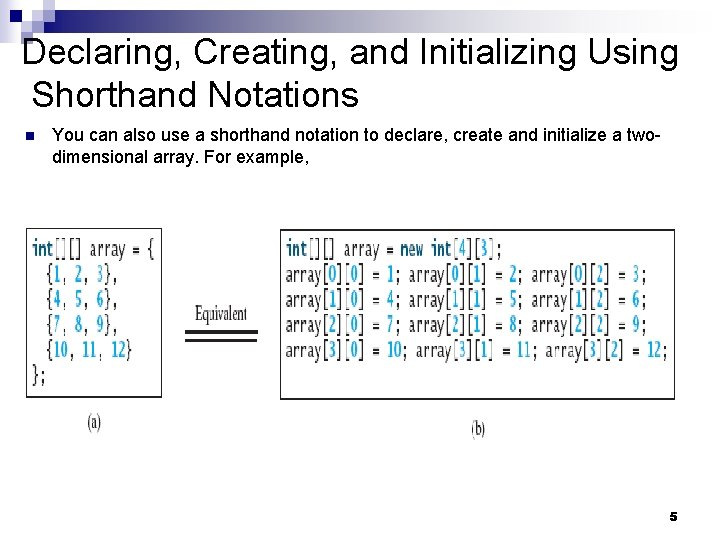Declaring, Creating, and Initializing Using Shorthand Notations n You can also use a shorthand notation to declare, create and initialize a twodimensional array. For example, 5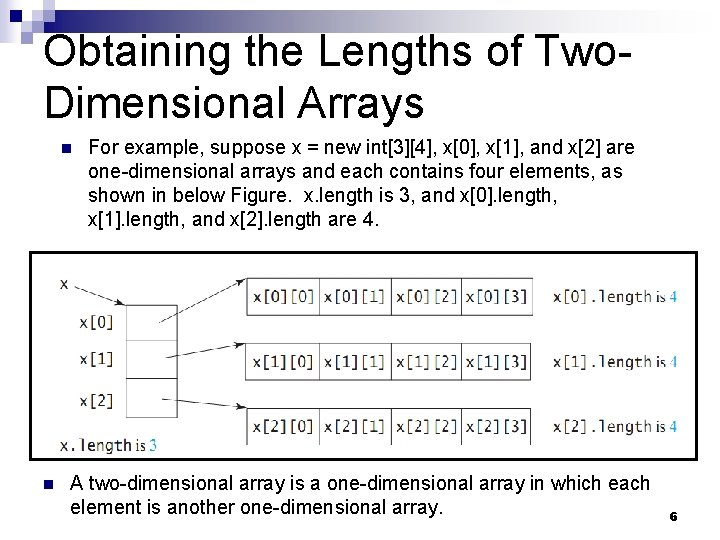Obtaining the Lengths of Two. Dimensional Arrays n n For example, suppose x = new int, x, x, and x are one-dimensional arrays and each contains four elements, as shown in below Figure. x. length is 3, and x. length, x. length, and x. length are 4. A two-dimensional array is a one-dimensional array in which each element is another one-dimensional array. 6Ragged Arrays n Each row in a two-dimensional array is itself an array. So, the rows can have different lengths. Such an array is known as a ragged array. For example: n As you can see, triangle. Array. length is 5, triangle. Array. length is 4, triangle. Array. length is 3, triangle. Array. length is 2, and triangle. Array. length is 1. 7Ragged Arrays, cont. n The same as before - you can create a ragged array using the following syntax: int[ ][ ] triangle. Array = new int [ ]; triangle. Array = new int; triangle. Array = new int; triangle. Array = new int; triangle. Array = new int; triangle. Array = new int; n n The syntax new int[ ] for creating an array requires the first index to be specified. The syntax new int[ ][ ] would be wrong. 8Processing Two-Dimensional Arrays Suppose an array matrix is created as follows: int[ ][ ] matrix = new int; n n The following loop initializes the array with user input values: 9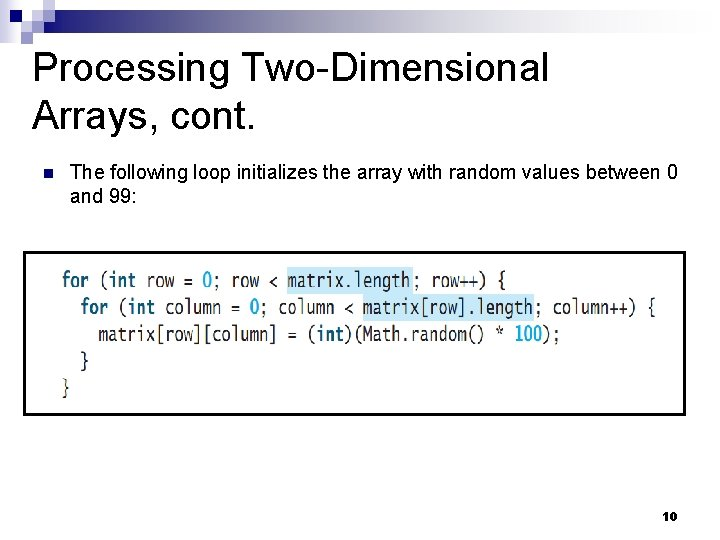Processing Two-Dimensional Arrays, cont. n The following loop initializes the array with random values between 0 and 99: 10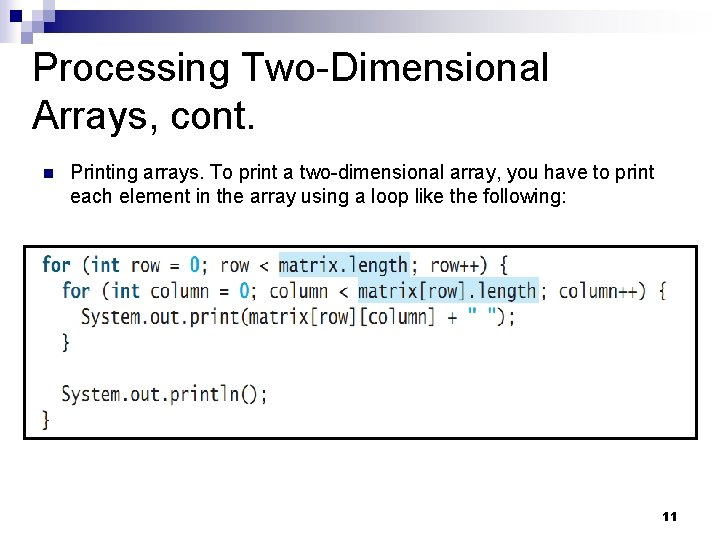Processing Two-Dimensional Arrays, cont. n Printing arrays. To print a two-dimensional array, you have to print each element in the array using a loop like the following: 11Processing Two-Dimensional Arrays, cont. n The following loop for summing elements by column in array. 12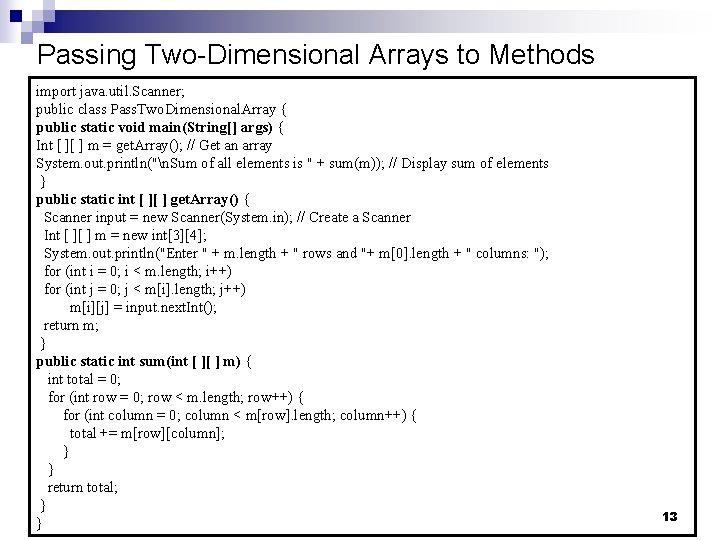Passing Two-Dimensional Arrays to Methods import java. util. Scanner; public class Pass. Two. Dimensional. Array { public static void main(String[] args) { Int [ ][ ] m = get. Array(); // Get an array System. out. println("n. Sum of all elements is " + sum(m)); // Display sum of elements } public static int [ ][ ] get. Array() { Scanner input = new Scanner(System. in); // Create a Scanner Int [ ][ ] m = new int; System. out. println("Enter " + m. length + " rows and "+ m. length + " columns: "); for (int i = 0; i < m. length; i++) for (int j = 0; j < m[i]. length; j++) m[i][j] = input. next. Int(); return m; } public static int sum(int [ ][ ] m) { int total = 0; for (int row = 0; row < m. length; row++) { for (int column = 0; column < m[row]. length; column++) { total += m[row][column]; } } return total; } } 13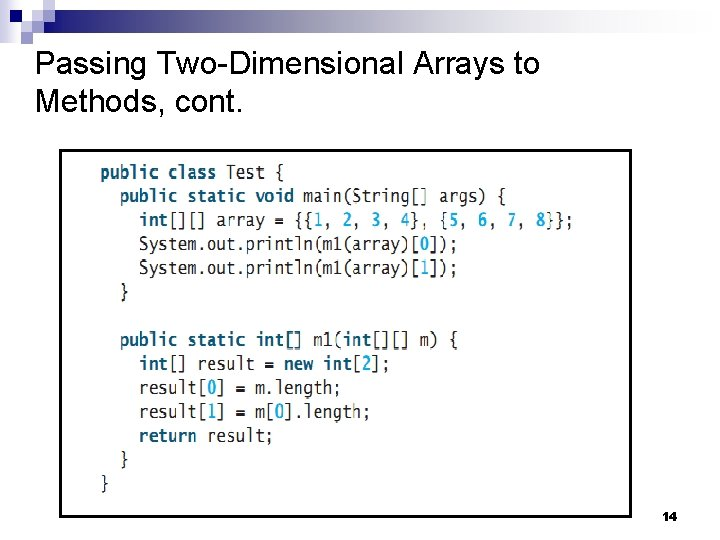Passing Two-Dimensional Arrays to Methods, cont. 14